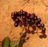首页   注册   登录
V2EX = way to explore
V2EX 是一个关于分享和探索的地方

V2EX  ›  LeetCode

# [笨方法学算法] 992-K 个不同整数的子数组 [算法求优化]

banxi1988 · 33 天前 · 593 次点击

## 原题

1 <= A.length <= 20000 1 <= A[i] <= A.length 1 <= K <= A.length

## 分类讨论，归纳总结

### K = 2

#### 只有一个最短子数组在两边:

1. [1,2] 解为 1，
2. [1,2,2] 解为 2.
3. [1,2,2,2] 的解为 3.
4. [1,1,1,2] 的解也为 3. 对于这种情况，我们显然可以总结出，对于子数组的个数，就是在满足要求的子数组的基础上不断的扩展以形成新的子数组。这种情况下子数组的计算。设，i,j-1 分别为最短子数组的下标。A[i:j] 为满足要求的最短子数组。数组长度为 n. 当 i = 0 或 j = n 时，解为 n-k + 1

#### 只有一个最短子数组在中间

1. [1,1,2,2] 解为 4,可以这样理解，除了中间最核心的最短子数组之外，其他的两边以排列组合来理解，可选可不选。左边多了一个 1，先与不先，两种选择，右边多了一个 2 也是两种选择，所以 2x2 =4.
2. [1,1,1,2,2,2] 解为 9，同上，左边 3 种选择，右边 3 种选择，3x3=9.
3. [1,1,1,1,2,2,2] 解为 12，同上左边 4 种选择，右边继续 3 种，4x3=12.

#### 有两个最短子数组的情况

1. [1,2,1] 解为 3. 以[1,2]为最短子数组为基础按上面的公式计算得 1x2 = 2,以第二个最短子数组 [2,1]为基础按上面的公式计算 2x1 = 2, 但是他们都共用了[1,2,1]这一个全数组作为子数组，所以总数是 2 + 2 -1 = 3

2. [1,1,2,1] 解为 5, 以[1,2]为基础子数组数为 2x2 =4,以 [2,1]为基础的子数组的个数也是 2x2=4,但是除了 [2,1] 这一基础子数组外，其他的子数组在以 [1,2] 为基础子数组的计算中出现过了。所以总的解为 4 + 1 =5

3. [1,1,2,1,1] 的解为 8 ,同上道理，以[1,2]为基础子数组的数为 2x3=6,以[2,1]为基础子数组的数为 2,因为以[2,1]为基础的子数组只能向右扩展也就是选择后面的 1，或者不选择。所以可选数为 2。所以总的解为 6 + 2=8

4. [1,1,2,2,1,1] 的解为 (2x4) + (2x2) = 12，以[2,1]为基础的最短子数组可以选择取或者不取左边 2，只要不取到左边的就 OK 了，因为子数组数是 2X2。

#### 有三个最短子数组的情况

1. [1,2,1,2] 的解为 (1x3) + (1x2) + (1x1) = 6
2. [1,1,2,1,2,2] 的解为 (2x4) + (1x3) + (1x2) = 13
3. [1,1,2,2,1,2,2] 的解为 (2x5) + (2x3) + (1x2) = 18

### K > 2 的情况

1. [1,2,3] 解为 1x1 =1
2. [1,1,2,3] 解为 2x1 = 2
3. [1,1,2,3,3] 解为 2x2 = 4
4. [1,1,2,3,1] 解为 2x2 + 1x1 = 5
5. [1,1,2,3,2,1] 解为 2x3 + 2x1 = 8

## 代码实现

43 / 55 个通过测试用例 状态：超出时间限制 提交时间：0 分钟之前

``````
import collections
from typing import List
class KRange:
def __init__(self,A:List[int],K:int):
self.A = A
self.K = K
self.alen = len(A)
self.num_counter = collections.Counter()
self.distinct_count = 0
self.i = 0
self.j = 0

def expand(self):
num = self.A[self.j]
self.j += 1
self.num_counter[num] += 1
if self.num_counter[num] == 1:
self.distinct_count += 1

def forward(self):
num = self.A[self.i]
self.i += 1
self.num_counter[num] -= 1
if self.num_counter[num] == 0:
self.distinct_count -= 1

def expand_to_max(self):
while self.can_expand():
self.expand()
return self.distinct_count == self.K

def can_expand(self):
if self.j < self.alen:
if self.distinct_count < self.K:
return True
num = self.A[self.j]
return self.num_counter[num] > 0
else:
return False

def can_move_forward(self):
return self.j < self.alen

def expand_to_K(self):
while self.distinct_count < self.K and self.j < self.alen:
self.expand()
self.trim_left()
return self.distinct_count == self.K

def trim_left(self):
# 1,1,2 这种 trim 成  1,2, 左边相同数去掉
if self.distinct_count == self.K:
while (self.i + 1) < self.j :
i = self.i
num = self.A[self.i]
if num == self.A[i+1]:
self.i += 1
self.num_counter[num] -= 1
else:
break

def range_slice(self):
return self.A[self.i:self.j]

class Solution:
def subarraysWithKDistinct(self, A: 'List[int]', K: 'int') -> 'int':
maxkrange = KRange(A=A,K=K)
total_count = 0
while maxkrange.can_move_forward():
if not maxkrange.expand_to_max():
break
n = maxkrange.j - maxkrange.i
prev_i = -1
minkrange = KRange(A=maxkrange.range_slice(),K=K)
while minkrange.expand_to_K():
count = (minkrange.i - (prev_i + 1) + 1) * (n - minkrange.j + 1)
total_count += count
prev_i = minkrange.i
minkrange.forward()
while maxkrange.distinct_count >= K:
maxkrange.forward()1 banxi1988   32 天前 感觉我应该在我之前的思路上进一步扩展，扩展到适应以多个数字的情况。或者根据 LeetCode 上的官方解答来改进思路。